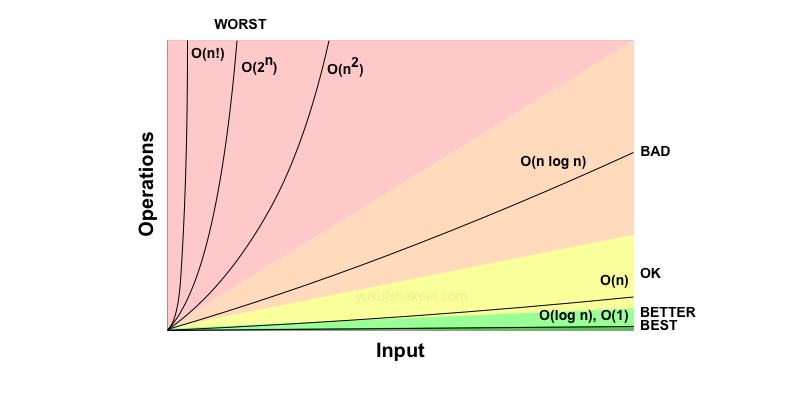# Time and Space complexity

In this post we will explore the time and space complexity of some of the important data structures and algorithms.

AlgorithmProgrammingData Structure

Welcome back to yet another blog post. Hope you are doing well. In this post I have written down the time and space complexity of different data structures and algorithms for reference.

log2n < n < nlog2n < n2 < 2n < n!

log2n is one of the best while n! is one of the worst.

## Time and Space complexity

Color code from best to worst complexity.

### Common Data Structures

WORST complexity

TimeSpace
Data StructureInsertDeleteSearchAccess
ArrayO(n)O(n)O(n)O(1)O(n)
StackO(1)O(1)O(n)O(n)O(n)
QueueO(1)O(1)O(n)O(n)O(n)
Binary Search TreeO(n)O(n)O(n)O(n)O(n)
AVL TreeO(log n)O(log n)O(log n)O(log n)O(n)
B TreeO(log n)O(log n)O(log n)O(log n)O(n)
Red Black TreeO(log n)O(log n)O(log n)O(log n)O(n)
Hash tableO(n)O(n)O(n)O(n)

### Array Sorting Algorithms

TimeSpace
AlgorithmBESTAVERAGEWORSTWORST
Bubble sortΩ(n)Θ(n2)O(n2)O(1)
Selection sortΩ(n2)Θ(n2)O(n2)O(1)
Insertion sortΩ(n)Θ(n2)O(n2)O(1)
Merge sortΩ(n log n)Θ(n log n)O(n log n)O(n)
Heap sortΩ(n log n)Θ(n log n)O(n log n)O(1)
Quick sortΩ(n log n)Θ(n log n)O(n2)O(log n)

## Growth rate

It is the rate at which the cost of the algorithm grows as the size of its input increases.

For example, consider the following code which prints the characters of a string.

``````str = "Hello World!";
for(i = 0; i < str.length; i++) {
print(str[i]);
}``````

There are total 12 characters in the above string `str`.

Now, let us look at the operations being performed in the above code snippet.

• The variable `str` is assigned the string value.
• The loop variable `i` is initialize with value `0`.
• The `for` loop condition is checked.
• If the condition is valid then the character is printed.
• After each loop the variable `i` is incremented by 1.
OperationCountNote
str = "Hello World!"1
i = 01
i < str.length13On the last i.e., 13th check the loop will exit.
print(str[i])12
i++12

Total operations is 1+1+13+12+12 i.e., 39 times.

If there are n characters in the string `str` then the growth rate of the above code will be the following.

OperationCountNote
str = string with n characters1
i = 01
i < str.lengthn+1On the last i.e., (n+1)th check the loop will exit.
print(str[i])n
i++n

So growth rate of the above code is 1+1+(n+1)+n+n i.e., 3n+3.

## Common growth rates

log2nnnlog2nn22nn!
0.0010.00121
1.0022.00442
1.5834.75986
2.0048.00161624
2.32511.612532120
3.321033.2210010243628800
4.322086.4440010485762.4329x1018
4.9130147.2190010737418242.65253x1032

From the above growth rate table we can safely say that log2n is the best while n! is the worst.

## Compare growth rates

In this section we will compare the growth rates and check with of the given functions is growing quicker.

To compare growth rates we can go through the following steps.

• Remove common values from both the functions.
• Take log of both the functions.
• Replace the input n with a very large value.
• Compare the value.

### Exercise 1

f(n) = n 3ng(n) = n 2nNote
n 3nn 2n
3n2nremoved common n
log23nlog22nlog of both functions
n log23n log22
log23log22removed common n
1.581

So, f(n) > g(n)

### Exercise 2

f(n) = n2g(n) = 2nNote
n22n
log2n2log22nlog of both functions
2 log2nn log22
2 log2nn
2 log2210210replacing n with 210
2x10 log22210
201024

So, f(n) < g(n)

### Exercise 3

f(n) = n2g(n) = (log2n)24Note
n2(log2n)24
log2n2log2(log2n)24log of both functions
2 log2n24 log2(log2n)
2 log221624 log2(log2216)replacing n with 216
2x16 log2224 log2(16 log22)
2x1624 log224
2x1624x4 log22
3296

So, for a smaller value of n we get f(n) < g(n).

Now, let us try for higher value of n.

f(n) = n2g(n) = (log2n)24Note
n2(log2n)24
log2n2log2(log2n)24log of both functions
2 log2n24 log2(log2n)
2 log22102424 log2(log221024)replacing n with 21024
2x1024 log2224 log2(1024 log22)
2x102424 log2210
2x102424x10 log22
2048240

So, for larger values of n we get f(n) > g(n).

## Determine time complexity

In this section we will find the time complexity of some of the code snippets.

### Example 1: loop

``````for(let i = 0; i < n; i++) {
console.log("Hello");
}``````

This code will execute n times so its time complexity is O(n).

### Example 2: nested loop

``````for(let i = 0; i < n; i++) {
for(let j = 0; j < n; j++) {
console.log("Hello");
}
}``````

The outer loop will execute n times. And for each outer loop execution the inner loop will execute n times. Therefore the total execution time is n2 and time complexity is O(n2).

### Example 3: nested loop

``````for(let i = 0; i < n; i++) {
for(let j = 0; j < i; j++) {
console.log("Hello");
}
}``````

The outer loop will execute n times.

During the first loop, when i=0, the inner loop will not execute. Then onwards, for every i, the inner loop will execute i-1 times.

So, total execution time is n(n-1) i.e., n2-n.

Therefore, the time complexity is O(n2).

### Example 3: logarithmic

``````for(let i = 1; i < n; i*=2) {
console.log("Hello");
}``````

From the above code, the value of i which increase like the following.

i = 1, 2, 4, 8, 16, ..., n

Or,

i = 20, 21, 22, ..., 2k

The loop will end when 2k = n.

Taking base 2 log of both sides we get log22k = log2n

Or,

k = log2n

The console.log statement will be executed log2n times.

So, time complexity of the above code snippet is O(log2n)